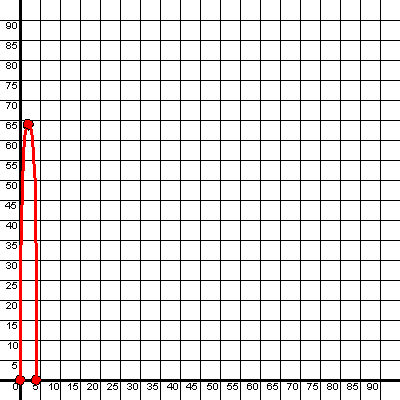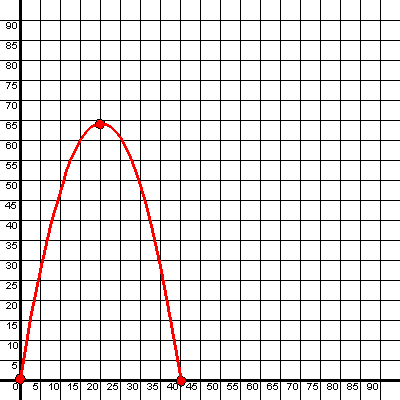Graph Scale Example

### Graph Scale Example:

The following graph shows the height, over time, of a ball thrown vertically at an initial speed of 64 ft./sec. The vertical axis is height above the ground in feet and the horizontal axis is time in seconds since the ball was thrown.Now, it is difficult to appreciate what is happening to the ball over time and there is a loss of precision with everything so crowded together.

Instead, let's scale the axes so the vertical axis is still height in feet but the horizontal axis represents time in tenths of a second. To achieve this I have to plot the vertex, for example, at the point (20,64) instead of the point (2,64):With this adjusted scale, it is easier to see the parabolic shape of the path of the ball over time.